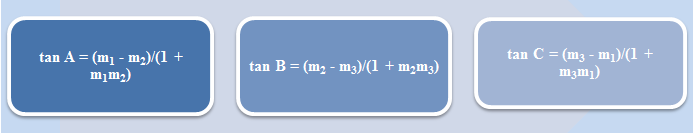×#### Thank you for registering.

One of our academic counsellors will contact you within 1 working day.

Click to Chat

1800-1023-196

+91-120-4616500

CART 0

• 0

MY CART (5)

Use Coupon: CART20 and get 20% off on all online Study Material

ITEM
DETAILS
MRP
DISCOUNT
FINAL PRICE
Total Price: Rs.

There are no items in this cart.
Continue Shopping```Angle between Pair of LinesStraight lines is an extremely important topic of IIT JEE Mathematics. It often fetches some direct questions in various competitions like the IIT JEE. Since the topic is quite vast, students are advised to spend sufficient time on grasping the various concepts. Angle between pair of straight lines is an important head under straight lines. We begin with the concept of angle between pair of lines and then discuss some of the illustrations on the same:

Angle between two Straight Lines

Suppose we have two straight lines y = m1x + c1 and y = m2x + c2, then the angle between these two lines is given by tan θ = |(m1 – m2)/ (1 + m1m2)|.

If m1, m2 and m3 are the slopes of three lines L1 = 0, L2 = 0 and L3 = 0, where m1 > m2 > m3 then the interior angles of the triangle ABC formed by these lines are given by,

tan A = (m1 - m2)/(1 + m1m2), tan B = (m2 - m3)/(1 + m2m3) and tan C = (m3 - m1)/(1 + m3m1).Remark:

If one of the line is parallel to y-axis then the angle between two straight lines is given by tan θ = ±1/m where ‘m’ is the slope of the other straight line.

If the two lines are a1x + b1y + c1 = 0 and a2x +  b2y + c2 = 0, then the formula becomes tan θ = |(a1b2 - b1a2)/(a1a2 + b1b2)|

Generally speaking, the angle between these two lines is assumed to be acute and hence, the value of tan θ is taken to be positive.

Watch this Video for more reference

Result:

Angle between pair of lines represented by ax2 + 2hxy + by2 = 0

Comparing the coefficients of x2, y2 and xy, we get

b(y – m1x) (y – m2x) = ax2 + 2hxy + by2

m1 + m2 = –2h/b and

m1 m2 = a/b

tan θacute = |(m2 – m1)/(1 + m1m2)|

= |√(m1–m2)2 – 4m1m2/(1 + m1m2)|

= |2√(h2 – ab)/(a + b)|

Note:

If two lines through the origin are represented by y = m1x and y = m2x, we cannot write

⇒ (y – m1x) (y – m2x) ≡ ax2 + 2hxy + by2

Because coefficient of y2 on left hand side is one on right hand side, it is b.

The given equation represents real lines only when h2 – ab > 0

If two lines are coincident then tan θ = 0 ⇒ h2 = ab

If two lines are perpendicular then m1m2 = 1 ⇒ a + b = 0

i.e. x2 + 2hxy – y2 always represents pair of mutually perpendicular lines through origin.

Two lines are equally inclined to axes but are not parallel:

For such a case let us take a line l1 which is inclined at an angle θ, then l2 is inclined at (π – θ).

tan (π – θ) = – tan θ which is the condition for two lines inclined equally to axes.

m1 = –h + √(h2–ab)/2 and m2 = –h – √(h2–ab)/2

Illustration:

What is the equation of the pair of lines through origin and perpendicular to ax2 + 2hxy + by2 = 0?

Solution:

Let ax2 + 2hxy + by2 = 0 represent the lines y = m1x (i) and y = m2x (ii)

Lines perpendicular to the lines (i) and (ii) are y = –1/m1 x and y = –1/m2 x respectively and passing through origin

i.e. m1y + x = 0 and m2y + x = 0

Their combined equation is given by

(m1y + x) (m2y + x) = 0

⇒ m1m2 y2 + (m1 + m2) xy + x2 = 0

⇒ a/2 y2 – 2h/b xy + x2 = 0

⇒ bx2 – 2hxy + ay2 = 0 is the equation of the pair of lines perpendicular to pair of lines ax2 + 2hxy + by2 = 0.

Illustration:

Find the angle between the lines represented by the equation 2x2 – 7xy + 3y2 = 0.

Solution:

The given equation is 2x2 – 7xy + 3y2 = 0.

This can be written as 2x2 – 7xy + 3y2 = (2x – y)(x-3y)

Therefore, the given lines are (2x – y) = 0 and (x-3y) = 0.

Now, here, a = 2, h = -7/2 and b = 3.

Hence, the angle between the lines is given by tan θ = 2√{(-7/2)2 – 6}/5 = 1.

Hence, θ = 45°.

Related Resources

For getting an idea of the type of questions asked, refer the previous year papers.

To read more, Buy study materials of Straight Lines comprising study notes, revision notes, video lectures, previous year solved questions etc. Also browse for more study materials on Mathematics here.
```### Course Features

• 731 Video Lectures
• Revision Notes
• Previous Year Papers
• Mind Map
• Study Planner
• NCERT Solutions
• Discussion Forum
• Test paper with Video Solution